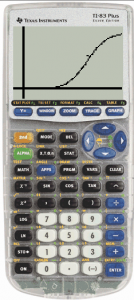# Logistics data and equation in TI-84+

///Logistics data and equation in TI-84+
Logistics data and equation in TI-84+ 2015-01-25T14:41:39+00:00Ebola Logistics data and equation in TI-84+